Operations on Spherical Functions edit page

The idea of variables of type S2Fun is to calculate with spherical functions similarly as Matlab does with vectors and matrices. In order to illustrate this we consider the following two spherical functions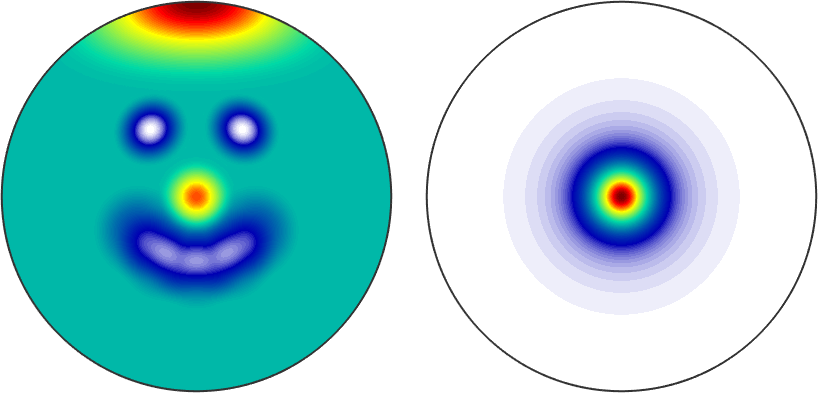## Basic arithmetic operations

Now the sum of these two spherical functions is again a spherical function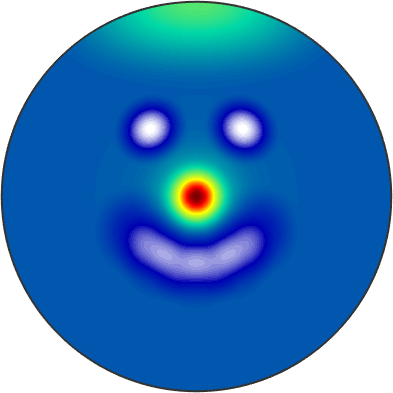Accordingly, one can use all basic operations like -, *, ^, /, min, max, abs, sqrt to calculate with variables of type S2Fun.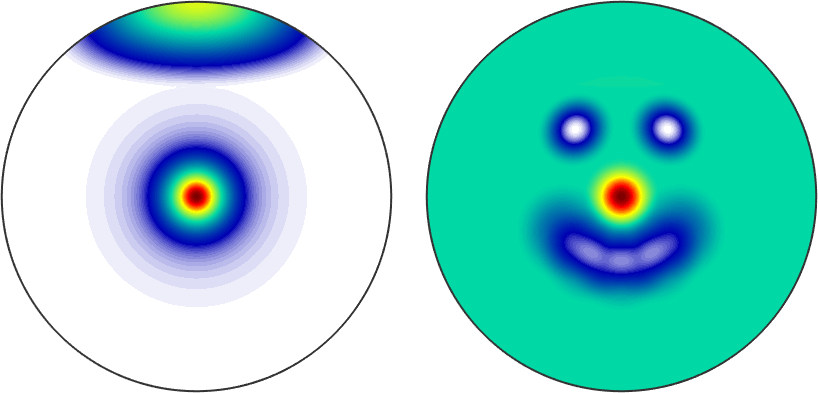## Local Extrema

The above mentioned functions min and max have very different use cases

• if two spherical functions are passed as arguments a spherical function defined as the pointwise min/max between these two functions is computed
• if a spherical function and a single number are passed as arguments a spherical function defined as the pointwise min/max between the function and the value is computed
• if only a single spherical function is provided the global maximum / minimum of the function is returned
• if additionally the option 'numLocal' is provided the certain number of local minima / maxima is computed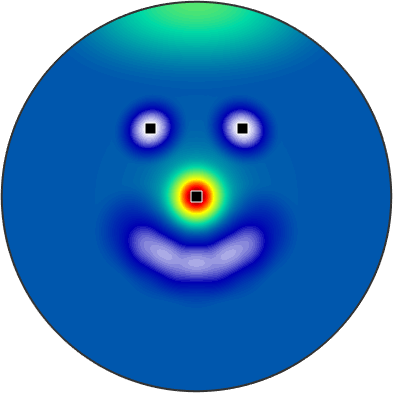## Integration

The surface integral of a spherical function can be computed by either mean or sum. The difference between both commands is that sum normalizes the integral of the identical function on the sphere to $$4 \pi$$, the command mean normalizes it to one. Compare

A practical application of integration is the computation of the $$L^2$$-norm which is defined for a spherical function $$f$$ by

$\| f \|_2 = \left(\int_{\mathrm{sphere}} \lvert f(\xi)\rvert^2 \,\mathrm d\xi\right)^{1/2}$

accordingly we can compute it by

or more efficiently by the command norm

## Differentiation

The differential of a spherical function in a specific point is a gradient, i.e., a three-dimensional vector which can be computed by the command grad

The gradients of a spherical function in all points form a spherical vector field and are returned by the function grad as a variable of type S2VectorFieldHarmonic.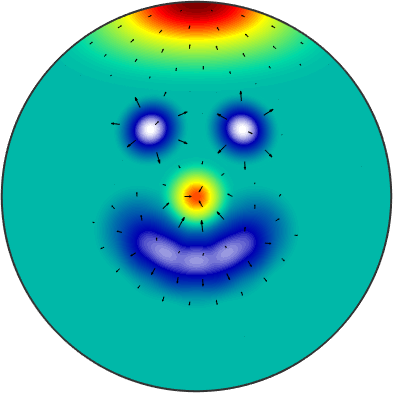We observe long arrows at the positions of big changes in intensity and almost invisible arrows in regions of constant intensity.

## Rotating spherical functions

Rotating a spherical function works with the command rotate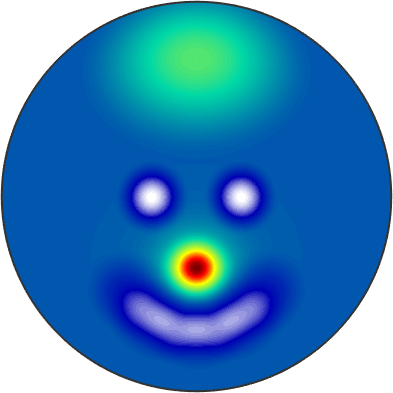A special case of rotation is symmetrysing it with respect to some symmetry. The following example symmetrises our smiley with respect to a two fold axis in $$z$$-direction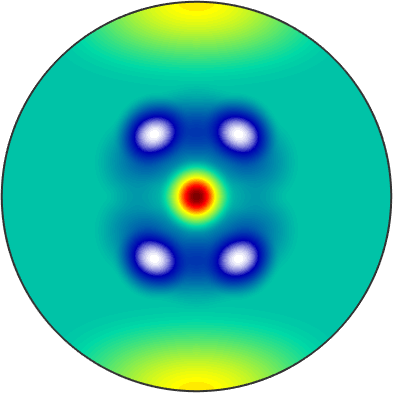The resulting function is of type S2FunHarmonicSym and knows about its symmetry.